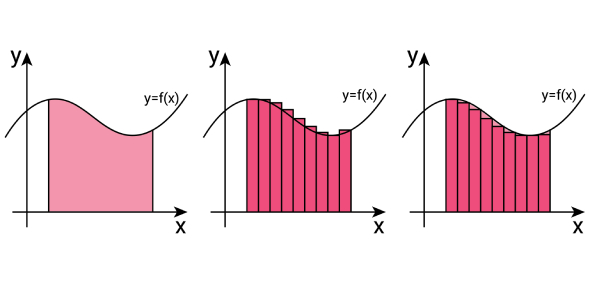# Quiz : How Much Do You Know About Functions And Function Notation

Approved & Edited by ProProfs Editorial Team
At ProProfs Quizzes, our dedicated in-house team of experts takes pride in their work. With a sharp eye for detail, they meticulously review each quiz. This ensures that every quiz, taken by over 100 million users, meets our standards of accuracy, clarity, and engagement.
| Written by Benlaing
B
Benlaing
Community Contributor
Quizzes Created: 1 | Total Attempts: 1,870
Questions: 10 | Attempts: 1,913SettingsFunctions are an important concept in mathematics. Just how much do you know about functions and function notation? If you think you know enough wouldn't it be fun to take a quiz and test your knowledge about it? Why not give it a try? Please make sure to read all the questions very carefully before answering. Interestingly, the quiz can help you prepare well for your upcoming examinations in a fun way. So, make sure to attempt all the questions. If you get stuck, you are allowed to refer to your solutions book for help Good Luck!

• 1.

### F(x) = 4x - 3             f(2)

• A.

7

• B.

2

• C.

5

• D.

None of the above

C. 5
Explanation
To find the value of f(2), we substitute 2 into the function f(x) = 4x - 3. Therefore, f(2) = 4(2) - 3 = 8 - 3 = 5.

Rate this question:

• 2.

### G(x) = 2x + 8             g(3)

• A.

14

• B.

12

• C.

12

• D.

None of the above

A. 14
Explanation
The given function g(x) is defined as 2x + 8. To find g(3), we substitute x = 3 into the function. Therefore, g(3) = 2(3) + 8 = 6 + 8 = 14.

Rate this question:

• 3.

### H(x) = - 3x + 2             h(7)

• A.

-21

• B.

-19

• C.

19

• D.

None of the above

B. -19
Explanation
To find the value of h(7), we substitute 7 into the function h(x). So, h(7) = -3(7) + 2 = -21 + 2 = -19. Therefore, the correct answer is -19.

Rate this question:

• 4.

### F(x) = - 2x + 4             f(- 3)

• A.

10

• B.

16

• C.

125

• D.

None of the above

A. 10
Explanation
To find the value of f(-3), we substitute -3 into the equation f(x) = -2x + 4. This gives us f(-3) = -2(-3) + 4 = 6 + 4 = 10. Therefore, the correct answer is 10.

Rate this question:

• 5.

### G(x) =             g(6)             11

• A.

X-6

• B.

X+11

• C.

X-5

• D.

None of the above

C. X-5
Explanation
The given function g(x) is equal to x-5. When g(6) is evaluated, it means that we substitute 6 into the function in place of x. Therefore, g(6) = 6-5 = 1. So, the correct answer is x-5.

Rate this question:

• 6.

### H(x)  =  5x - 4             h()             36

• A.

8

• B.

9

• C.

12

• D.

None of the above

A. 8
Explanation
The question is asking for the value of h() when the input is 36. The function h(x) is defined as 5x - 4. To find h(36), we substitute 36 into the function and evaluate it. Therefore, h(36) = 5(36) - 4 = 180 - 4 = 176. However, none of the answer choices provided match this result, so the correct answer is not available.

Rate this question:

• 7.

### Evaluate f(-2) if f(x) = x2+ 3

• A.

-1

• B.

1

• C.

7

• D.

-7

C. 7
Explanation
To evaluate f(-2), we substitute -2 for x in the given function f(x) = x^2 + 3. Thus, f(-2) = (-2)^2 + 3 = 4 + 3 = 7. Therefore, the correct answer is 7.

Rate this question:

• 8.

### Given f(x) = -4x - 10 and f(x) = 10. Find x

• A.

X = -5

• B.

X = 5

• C.

X = -50

• D.

X = 30

A. X = -5
Explanation
To find the value of x, we need to equate the given function f(x) to 10 and solve for x. By substituting 10 for f(x) in the equation -4x - 10 = 10, we get -4x = 20. Dividing both sides by -4, we find x = -5. Therefore, the correct answer is x = -5.

Rate this question:

• 9.

### Evaluate f(-2) if f(x) = -1/2x + 3

• A.

2

• B.

-2

• C.

-1

• D.

4

D. 4
Explanation
To evaluate f(-2), we substitute -2 into the function f(x) = -1/2x + 3. So, f(-2) = -1/2(-2) + 3 = 1 + 3 = 4.

Rate this question:

• 10.

### What is the range of the following relation: (9, -2) (4, 3)  ( 8, 10) ( -4, 8)

• A.

-2, 3, 8, 10

• B.

(9, -2) ( 4, 3)

• C.

(8, 10) (-4, 8)

• D.

-4, 4, 8, 9Back to top